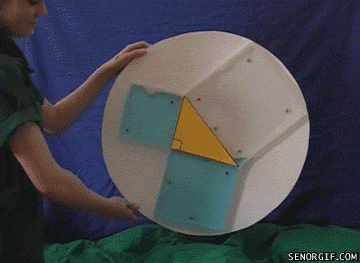### Pythagorean Theorem

In: Science

18 Dec 2012

Sometimes the best way to explain something is to demonstrate it.## 15 Responses to Pythagorean TheoremChaX

All teaching should be practical, like this!Jim

This is an interesting physical demonstration, but it offers no insight as to why the Pythagorean Theorem is true.SOB

@Jim
You must be kidding.
Ignoring the fact that these are 3D cubes with volumes of water. Look at it in 2D and look at the Pythagorean Theorem. It’s a great demonstration.Zorzal

@SOB: Actually, it’s NOT a demonstration (in the scientific, formal sense). For example, the theorem could be wrong by a 1% or so, and you would not notice it from this visual. @Jim is right in the sense that there is no formally correct series of statements proving the theorem.

It is however, a very cool (and known for many years) exercise. Old math teachers (me included) used to be do the trick with sand.Blorgon

@Zorzal what criteria is needed to constitute a “scientific, formal proof” in your understanding? I’ve seen a number of mathematical proofs for the pythagorean theorem that all seem grounded in sound logical reasoning. Granted, without an explanation behind it this gif is simply a demonstration of why the pythagorean theorem works, but there are a few proofs as well.Tim Erickson

Maybe Zorzal would be happier calling this an “illustration.” And it’s a good one, though it could be off by 1%. As Blorgon points out, there are LOTS of formal proofs. But Jim is right: it doesn’t tell us why it works — it only shows us (very effectively) that it seems to work for this particular triangle.

I recently gave an assignment to preservice teachers with seven or so URLs for youtube videos about the Pythagorean Theorem. The discussion about which were proofs and which were demonstrations, and how we might use them with students, was really useful.Eyeyeyeyey

¡Viva Pitágoras!Michiel

I’d rather see a useful example of how and why we would use the pythagoream theorem in the real world. Let’s say you need to calculate a distance on a map e.g.

Without that useful problem to solve the pythagoream theorem is nothing but a silly game or puzzle. Granted, some students like puzzles, but most are bored to death by anything math related.

It’s better to have an actual treasure hunt, where the ones who solve the equations are the fastest to get to the treasure.Miguel

I agree, note this only should proove it’s true for this triangle, not for all the rest. But I love it and will show it to my students.KRS

How can one know that all three of those cuboids have the same height?Cinek

be koz C^2=a^2+b^2Goras

Great presentation! Really useful for the teachers.

Chujnia na maksa. Wykop Kurwa.snp

@Goras Great=Chujnia? the teachers=Kurwa? You sabotage Google Translate ;PMArian

Fajnie kurwa, ale tu jest internet i tu się komentuje po polsku 🙂Steven

@Jim: that’s why it says “demonstration”. Nobody pretends it’s “proof”.